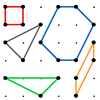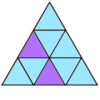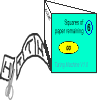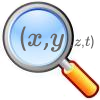# Working Systematically

Working Systematically is part of our Working Mathematically collection.

#### Scroll down to see our complete collection of problems that require students to work systematically, or explore the two sub-collections focussing on important aspects of systematic working.### Noticing Patterns

The key to solving these problems is to notice patterns or properties. Encouraging students to organise their work systematically allows them to notice what might not otherwise be obvious.### Finding All Solutions

These problems challenge students to find all possible solutions. One of the best answers to "How do you know you have found them all" is to be able to say "I worked systematically!"### Summing Consecutive Numbers

##### Age 11 to 14Challenge Level

This problem offers a simple context for students to explore, make generalisations and prove conjectures, working numerically and algebraically.### M, M and M

##### Age 11 to 14Challenge Level

This problem offers the students an opportunity to consolidate what they are expected to know about mean, mode and median whilst also challenging them to work systematically, and justify their reasoning##### Age 11 to 14Challenge Level

This problem is a good activity for the visualisation of symmetry, and for encouraging students to work systematically.### Can They Be Equal?

##### Age 11 to 14Challenge Level

Sometimes area and perimeter of rectangles are taught separately, and are often confused. In this problem students consider the relationship between them.##### Age 11 to 14Challenge Level

By selecting digits for an addition grid, what targets can you make?### Sticky Numbers

##### Age 11 to 14Challenge Level

Can you arrange the numbers 1 to 17 in a row so that each adjacent pair adds up to a square number?### Isosceles Triangles

##### Age 11 to 14Challenge Level

This problem encourages students to use coordinates, area and isosceles triangles to solve a non-standard problem. To find all possible solutions they will need to work systematically.### Shifting Times Tables

##### Age 11 to 14Challenge Level

This problem encourages students to think about the properties of numbers. It could be used as an introduction to work on linear sequences and straight line graphs.### Gabriel's Problem

##### Age 11 to 14Challenge Level

Gabriel multiplied together some numbers and then erased them. Can you figure out where each number was?### American Billions

##### Age 11 to 14Challenge Level

This is an engaging context in which to reinforce rules of divisibility and challenge students to reason mathematically and work systematically.### ACE, TWO, THREE...

##### Age 11 to 14Challenge Level

The engaging nature of this trick means that students are often prepared to persevere on this task. It may offer a chance to nurture a sense of resilience amongst your students.### Peaches Today, Peaches Tomorrow...

##### Age 11 to 14Challenge Level

This problem could replace repetitive textbook work on calculating fractions of integers. It offers plenty of practice of these calculations while requiring students to come up with problem-solving strategies.### 1 Step 2 Step

##### Age 11 to 14Challenge Level

This problem is inaccessible without looking at simpler cases, and thus helps students to see the value of specialising in order to generalise.### Working Systematically - Short Problems

##### Age 11 to 16

A collection of short Stage 3 and 4 problems on Working Systematically.### Charlie's Delightful Machine

##### Age 11 to 16Challenge Level

This problem could be used to reinforce work on recording and describing linear sequences.### Product Sudoku

##### Age 11 to 16Challenge Level

This problem offers an engaging context in which to apply knowledge of factors, multiples and prime factor decomposition.### Nine Colours

##### Age 11 to 16Challenge Level

Can you use small coloured cubes to make a 3 by 3 by 3 cube so that each face of the bigger cube contains one of each colour?### Two and Two

##### Age 11 to 16Challenge Level

How many solutions can you find to this sum? Each of the different letters stands for a different number.### What's Possible?

##### Age 14 to 16Challenge Level

As well as introducing the difference of two squares, this problem allows students to explore, conjecture and use algebra to justify their results.### Pick's Theorem

##### Age 14 to 16Challenge Level

This problem allows students to consolidate their understanding of how to calculate the area of irregular shapes, while offering an opportunity to explore and discover an interesting result.### Sorted

##### Age 14 to 18Challenge Level

This problem uses playing cards and video to introduce students to some well-known sorting algorithms.### A Long Time at the Till

##### Age 14 to 18Challenge Level

This problem provides an introduction to advanced mathematical behaviour which might not typically be encountered until university. The content level is secondary, but the thinking is sophisticated and will benefit the mathematical development of school-aged mathematicians.### Ante Up

##### Age 16 to 18Challenge Level

Use cunning to work out a strategy to win this game.### Stage 5 Cipher Challenge

##### Age 16 to 18Challenge Level

Can you crack these very difficult challenge ciphers? How might you systematise the cracking of unknown ciphers?